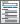Home  /  Products  /  Features  /  ANOVA / MANOVA

## ANOVA / MANOVA

Fit one- and two-way models. Or fit models with three, four, or even more factors. Analyze data with nested factors, with fixed and random factors, or with repeated measures. Use ANCOVA models when you have continuous covariates and MANOVA models when you have multiple outcome variables. Further explore the relationships between your outcome and predictors by estimating effect sizes and computing least-squares and marginal means. Perform contrasts and pairwise comparisons. Analyze and plot interactions. And much more.
• Eta-squared—η2
• Epsilon-squared—ε2
• Omega-squared—ω2
• Confidence intervals

Postestimation after ANOVA

Postestimation after MANOVA

• View and run all postestimation features for your command
• Automatically updated as estimation commands are run
• Automatically create indicators based on categorical variables
• Form interactions among discrete and continuous variables
• Include polynomial terms
• Perform contrasts of categories/levels
Watch Introduction to factor variables in Stata tutorials
Watch Introduction to margins in Stata tutorials
Watch Profile plots and interaction plots in Stata tutorials
• Analysis of main effects, simple effects, interaction effects, partial interaction effects, and nested effects
• Comparisons against reference groups, of adjacent levels, or against the grand mean
• Orthogonal polynomials
• Helmert contrasts
• Custom contrasts
• ANOVA-style tests
• Contrasts of nonlinear responses
• Balanced and unbalanced data
• Contrasts of means, intercepts, and slopes
• Graphs of contrasts• Compare estimated means, intercepts, and slopes
• Compare marginal means, intercepts, and slopes
• Balanced and unbalanced data
• Nonlinear responses
• Multiple-comparison adjustments: Bonferroni, Šidák, Scheffé, Tukey HSD, Duncan, and Student–Newman–Keuls adjustments
• Group comparisons that are significant
• Graphs of pairwise comparisons